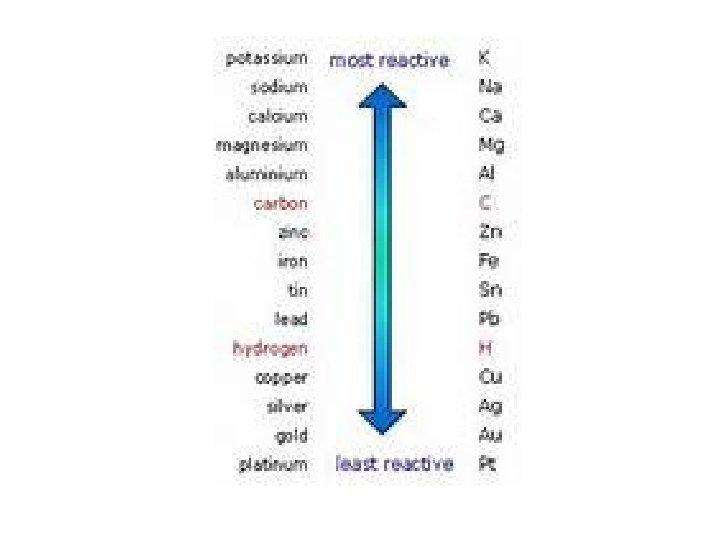# Chemical Reactions 1 Types of Reactions 2 Indications

• Slides: 12Chemical Reactions 1. Types of Reactions 2. Indications of Reactions 3. Relevance of Chemical Equations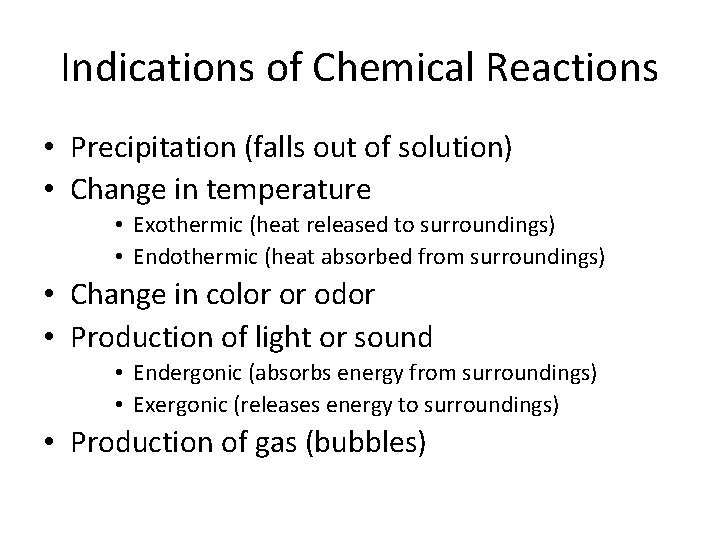Indications of Chemical Reactions • Precipitation (falls out of solution) • Change in temperature • Exothermic (heat released to surroundings) • Endothermic (heat absorbed from surroundings) • Change in color or odor • Production of light or sound • Endergonic (absorbs energy from surroundings) • Exergonic (releases energy to surroundings) • Production of gas (bubbles)Types of Chemical Reactions • Synthesis: A + B AB 2 Hg + O 2 2 Hg. O • Decomposition: AB A + B • Single-displacement: X + AB XB + A • Cu + Ag. NO 3 Cu. NO 3 + Ag • Double-displacement: XY + AB XB + AY • HCl + Na. OH Na. Cl + H 2 O • Combustion: XY + O 2 different products • CH 2 O + O 2 CO 2 + H 2 OSynthesis Double-displacement Single-displacement Oxidation/ synthesis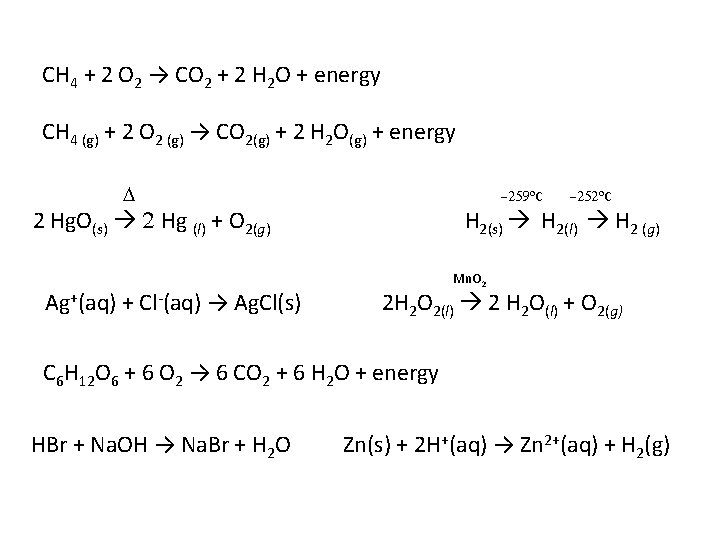CH 4 + 2 O 2 → CO 2 + 2 H 2 O + energy CH 4 (g) + 2 O 2 (g) → CO 2(g) + 2 H 2 O(g) + energy D -259 o. C 2 Hg. O(s) 2 Hg (l) + O 2(g) Ag+(aq) + Cl-(aq) → Ag. Cl(s) -252 o. C H 2(s) H 2(l) H 2 (g) Mn. O 2 2 H 2 O 2(l) 2 H 2 O(l) + O 2(g) C 6 H 12 O 6 + 6 O 2 → 6 CO 2 + 6 H 2 O + energy HBr + Na. OH → Na. Br + H 2 O Zn(s) + 2 H+(aq) → Zn 2+(aq) + H 2(g)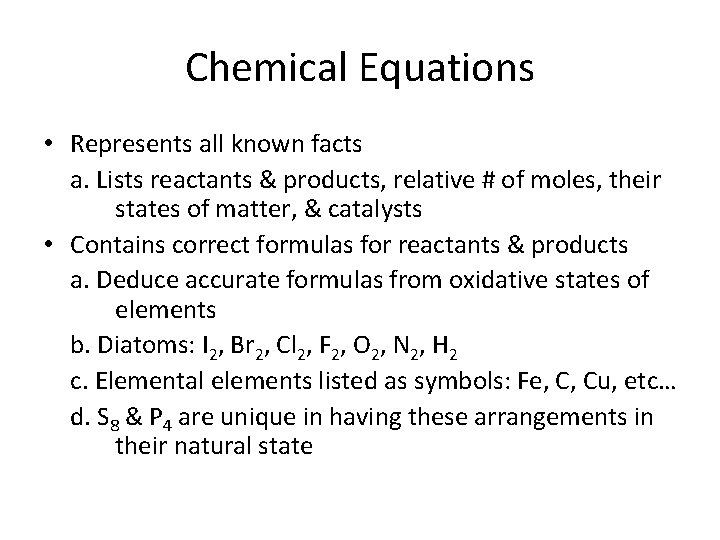Chemical Equations • Represents all known facts a. Lists reactants & products, relative # of moles, their states of matter, & catalysts • Contains correct formulas for reactants & products a. Deduce accurate formulas from oxidative states of elements b. Diatoms: I 2, Br 2, Cl 2, F 2, O 2, N 2, H 2 c. Elemental elements listed as symbols: Fe, C, Cu, etc… d. S 8 & P 4 are unique in having these arrangements in their natural state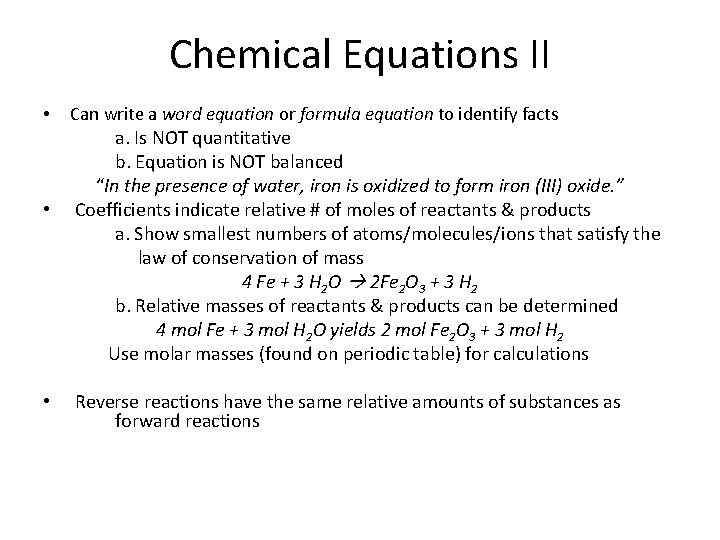Chemical Equations II • • • Can write a word equation or formula equation to identify facts a. Is NOT quantitative b. Equation is NOT balanced “In the presence of water, iron is oxidized to form iron (III) oxide. ” Coefficients indicate relative # of moles of reactants & products a. Show smallest numbers of atoms/molecules/ions that satisfy the law of conservation of mass 4 Fe + 3 H 2 O 2 Fe 2 O 3 + 3 H 2 b. Relative masses of reactants & products can be determined 4 mol Fe + 3 mol H 2 O yields 2 mol Fe 2 O 3 + 3 mol H 2 Use molar masses (found on periodic table) for calculations Reverse reactions have the same relative amounts of substances as forward reactions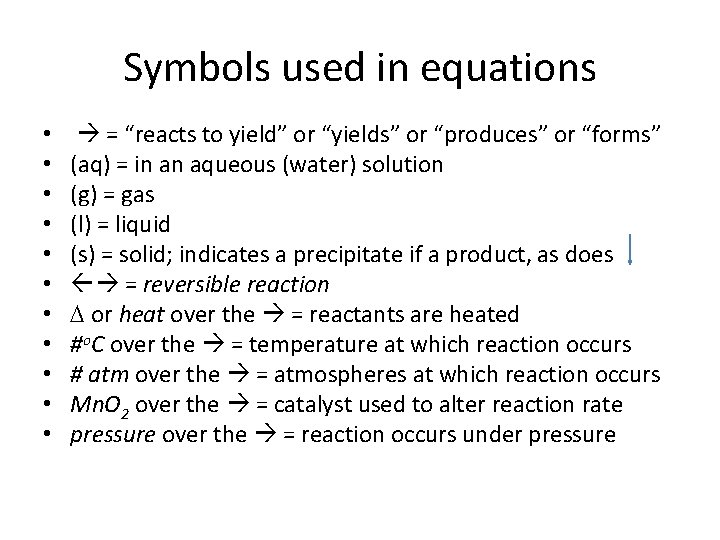Symbols used in equations • • • = “reacts to yield” or “yields” or “produces” or “forms” (aq) = in an aqueous (water) solution (g) = gas (l) = liquid (s) = solid; indicates a precipitate if a product, as does = reversible reaction D or heat over the = reactants are heated #o. C over the = temperature at which reaction occurs # atm over the = atmospheres at which reaction occurs Mn. O 2 over the = catalyst used to alter reaction rate pressure over the = reaction occurs under pressureBalancing Chemical Equations • Balance equation according to law of conservation of mass – One can only change coefficients, NEVER subscripts – Balance different types of atoms one at a time – Balance those in compounds 1 st – Balance polyatomic ions that appear on both sides of the equation – Balance free H & O atoms last. • ALWAYS do a final count of all atoms to ensure equation is balanced.Activity Series of Metals • Most reactive metal at top of list can replace those below it. • Reactions based on empirical data • Chemical equations don’t always relate to real-world occurrences. • Timing is everything. (It may happen so slowly that you don’t live long enough to see it. ) • Temperature may be a factor.